# How to Calculate and Solve for Gravity Anomaly for a Semi-Infinite Horizontal Sheet | Gravity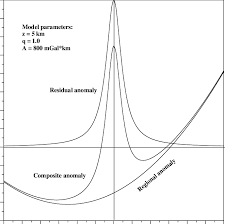The image above represents gravity anomaly for a semi-infinite horizontal sheet.

To compute for gravity anomaly for a semi-infinite horizontal sheet, five essential parameters are needed and these parameters are Gravitational Constant (G), Anomalous Density (Δρ), Thickness of the Sheet (t), Horizontal Distance (x) and Depth Buried (d).

The formula for calculating gravity anomaly for a semi-infinite horizontal sheet:

δgz = 2GΔρt [π/2 + tan-1(x/d)]

Where:

δgz = Gravity Anomaly for a Semi-Infinite Horizontal Sheet
G = Gravitational Constant
Δρ = Anomalous Density
t = Thickness of the Sheet
x = Horizontal Distance
d = Depth Buried

Let’s solve an example;
Find the gravity anomaly for a semi-infinite horizontal sheet when the gravitational constant is 6.67E-11, the anomalous density is 12, the thickness of the sheet is 14, the horizontal distance is 18 and the depth buried is 5.

This implies that;

G = Gravitational Constant = 6.67E-11
Δρ = Anomalous Density = 12
t = Thickness of the Sheet = 14
x = Horizontal Distance = 18
d = Depth Buried = 5

δgz = 2GΔρt [π/2 + tan-1(x/d)]
δgz = 2(6.67e-11)(12)(14) [π/2 + tan-1(18/5)]
δgz = 2.2411200000000002e-8 [1.570 + tan-1(3.6)]
δgz = 2.2411200000000002e-8 [1.570 + 74.475]
δgz = 2.2411200000000002e-8 [76.046]
δgz = 0.000001704

Therefore, the gravity anomaly for a semi-infinite horizontal sheet is 0.000001704 mGal.

Nickzom Calculator – The Calculator Encyclopedia is capable of calculating the gravity anomaly for a semi-infinite horizontal sheet.

To get the answer and workings of the gravity anomaly for a semi-infinite horizontal sheet using the Nickzom Calculator – The Calculator Encyclopedia. First, you need to obtain the app.

You can get this app via any of these means:

To get access to the professional version via web, you need to register and subscribe for NGN 2,000 per annum to have utter access to all functionalities.
You can also try the demo version via https://www.nickzom.org/calculator

Apple (Paid) – https://itunes.apple.com/us/app/nickzom-calculator/id1331162702?mt=8
Once, you have obtained the calculator encyclopedia app, proceed to the Calculator Map, then click on Geology under Add-on.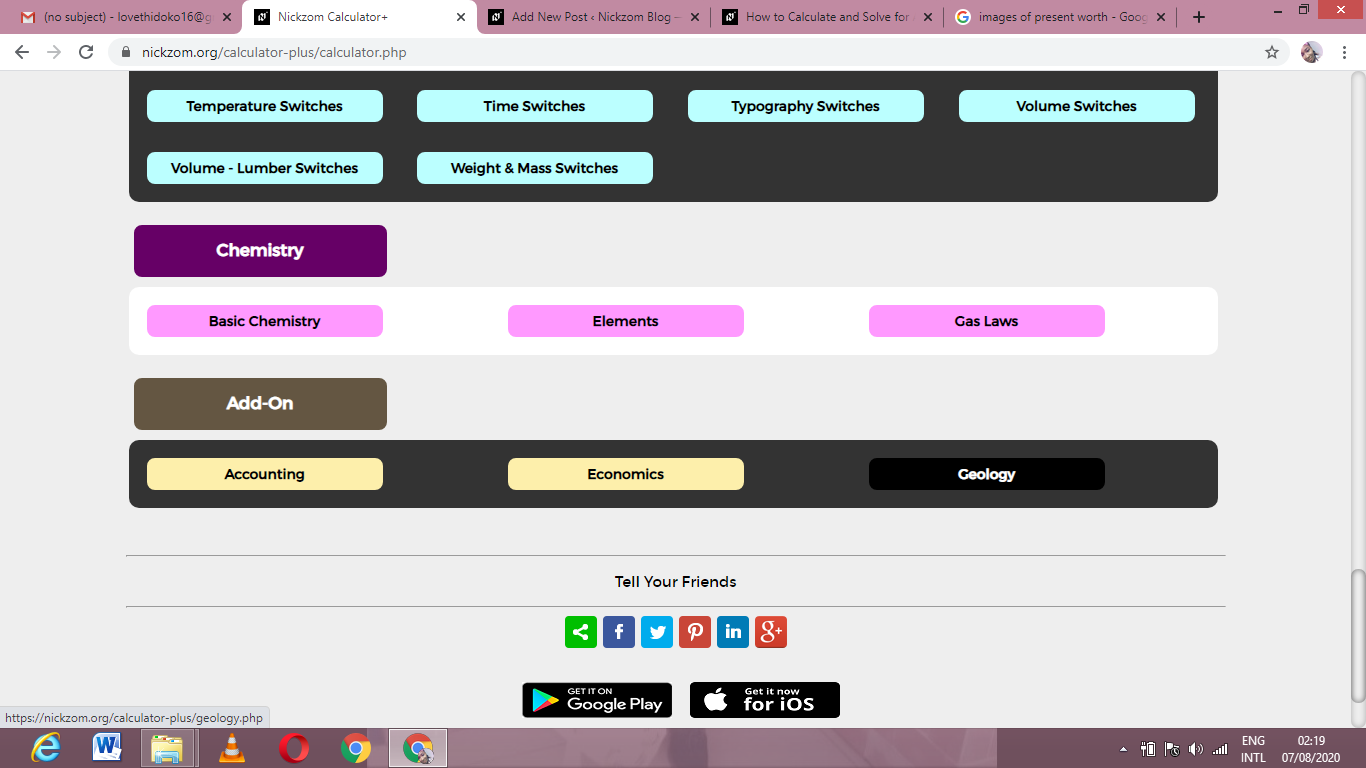Now, Click on Gravity under Geology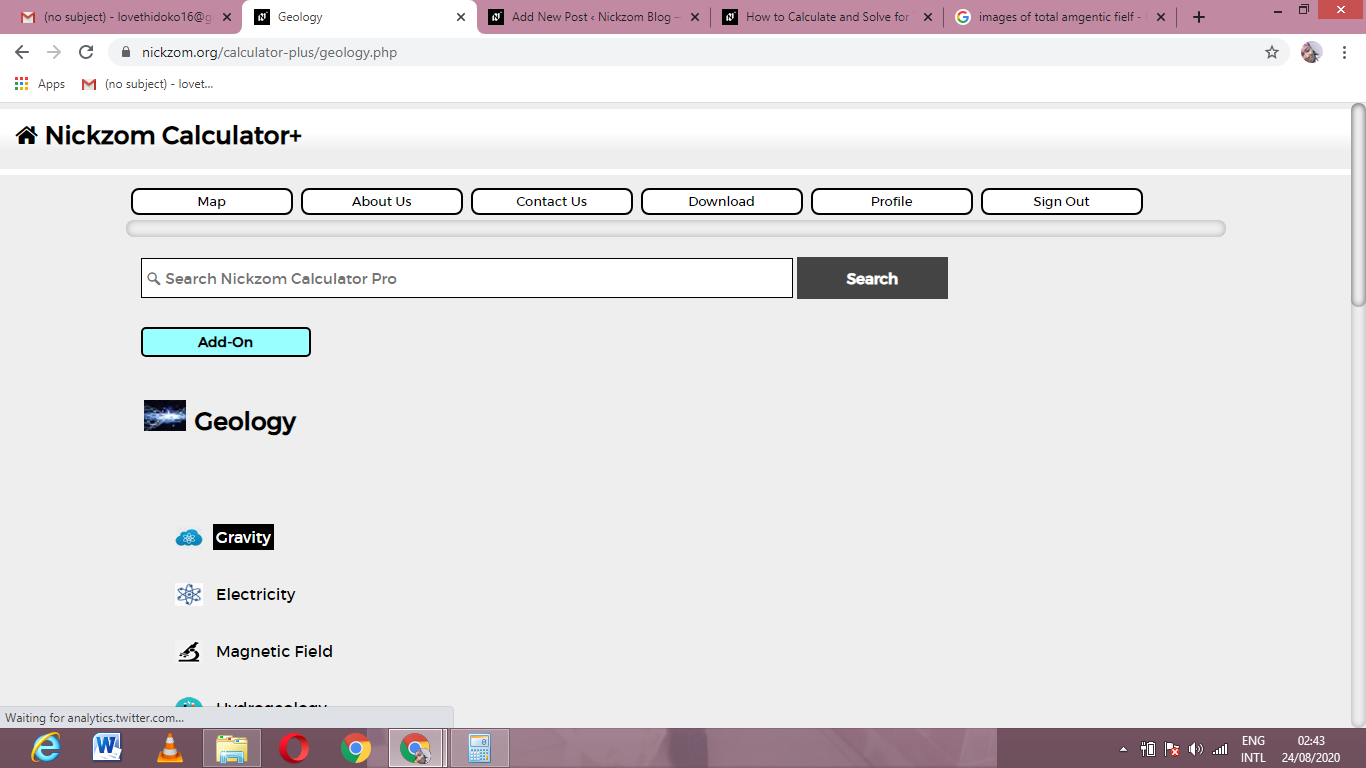Now, Click on gravity anomaly for a semi-infinite horizontal sheet under Gravity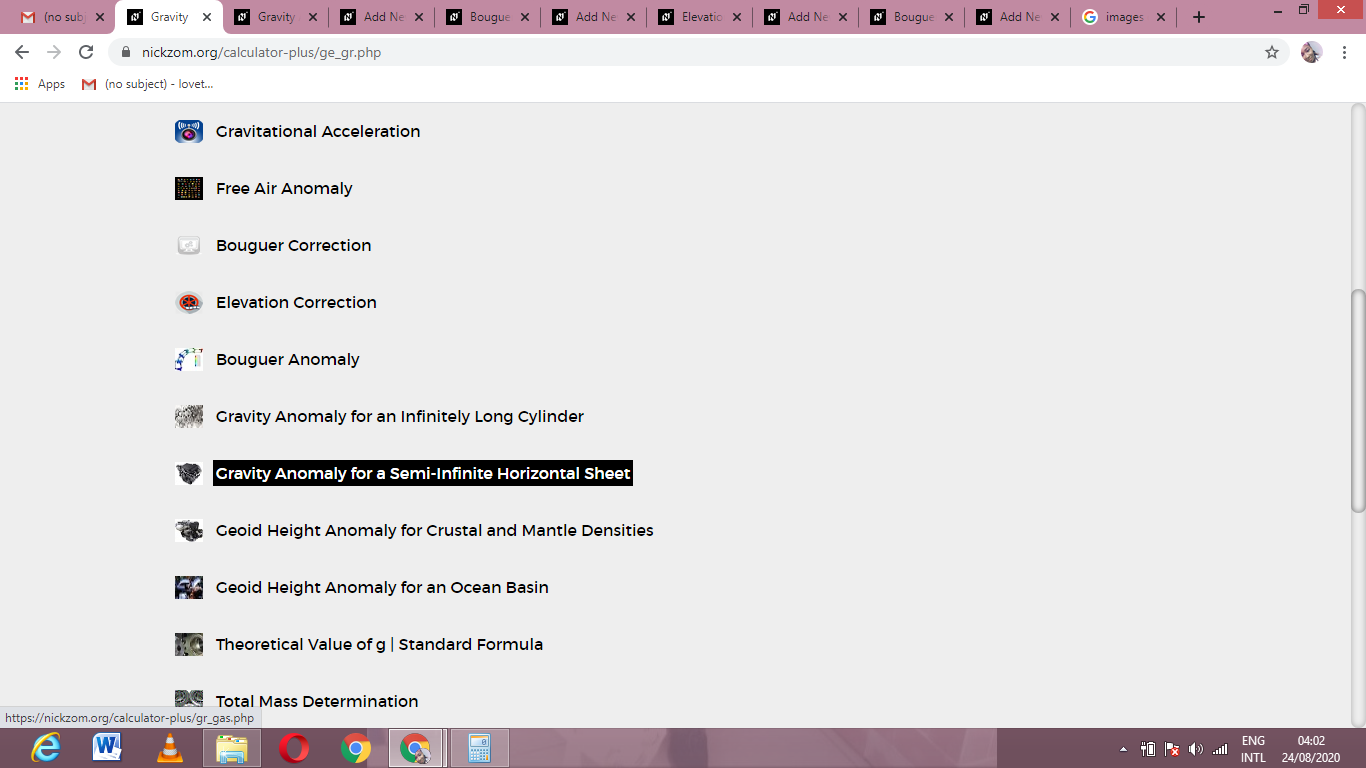The screenshot below displays the page or activity to enter your values, to get the answer for the gravity anomaly for a semi-infinite horizontal sheet according to the respective parameters which are the Gravitational Constant (G), Anomalous Density (Δρ), Thickness of the Sheet (t), Horizontal Distance (x) and Depth Buried (d).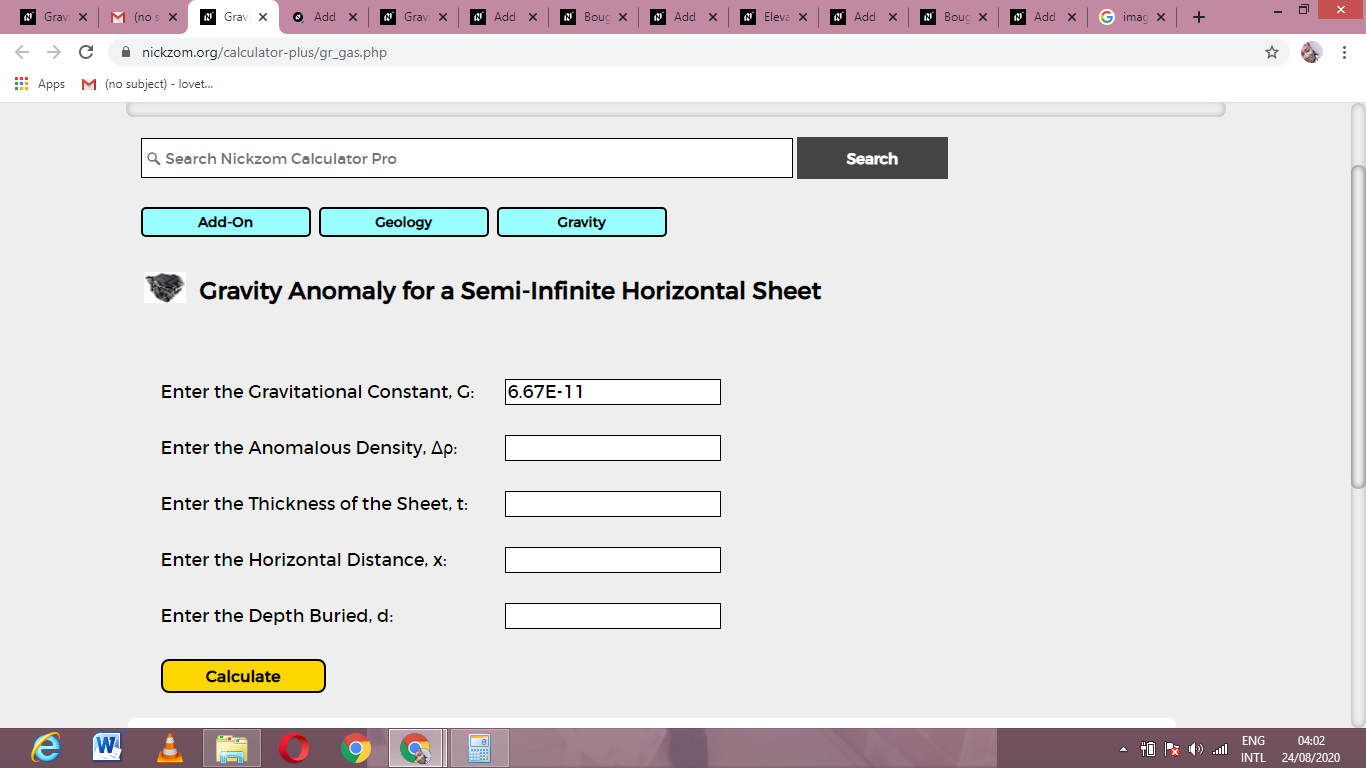Now, enter the values appropriately and accordingly for the parameters as required by the Gravitational Constant (G) is 6.67E-11, Anomalous Density (Δρ) is 12, Thickness of the Sheet (t) is 14, Horizontal Distance (x) is 18 and Depth Buried (d) is 5.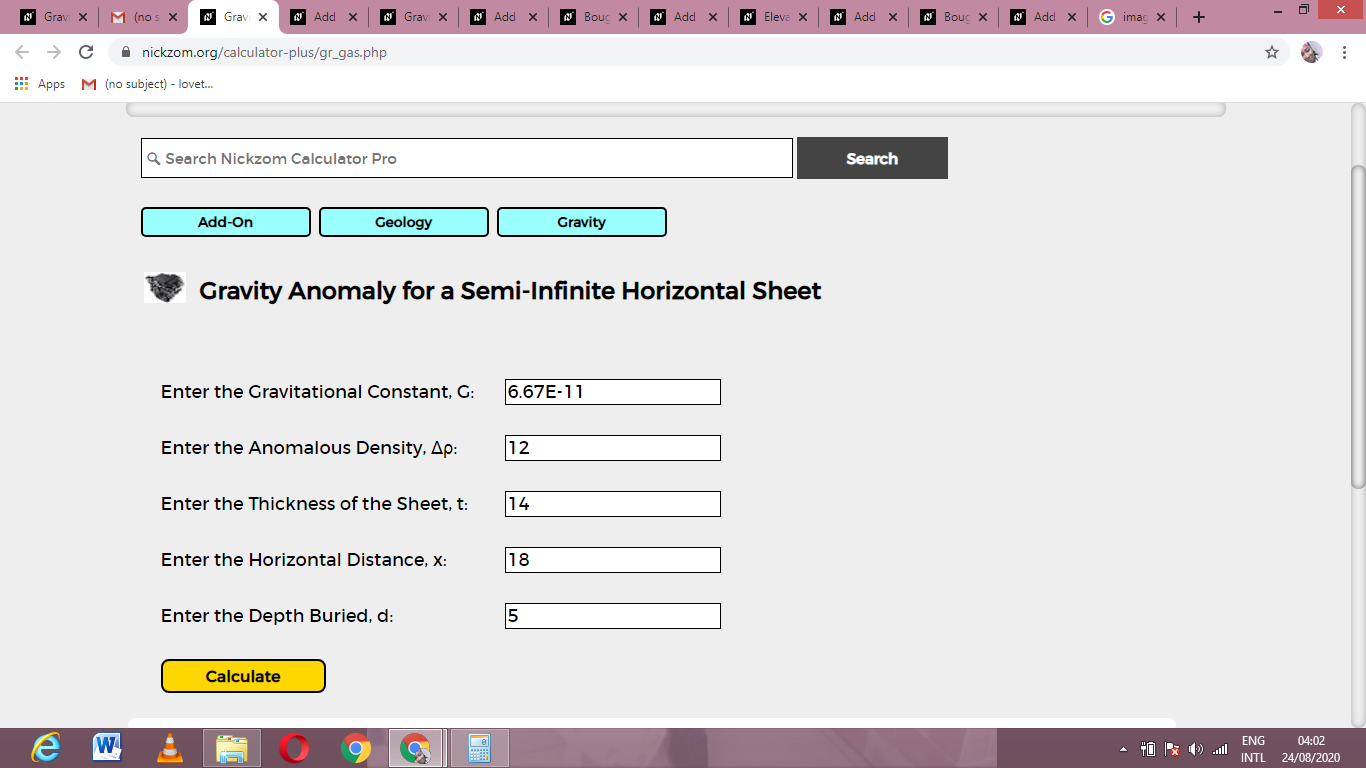Finally, Click on Calculate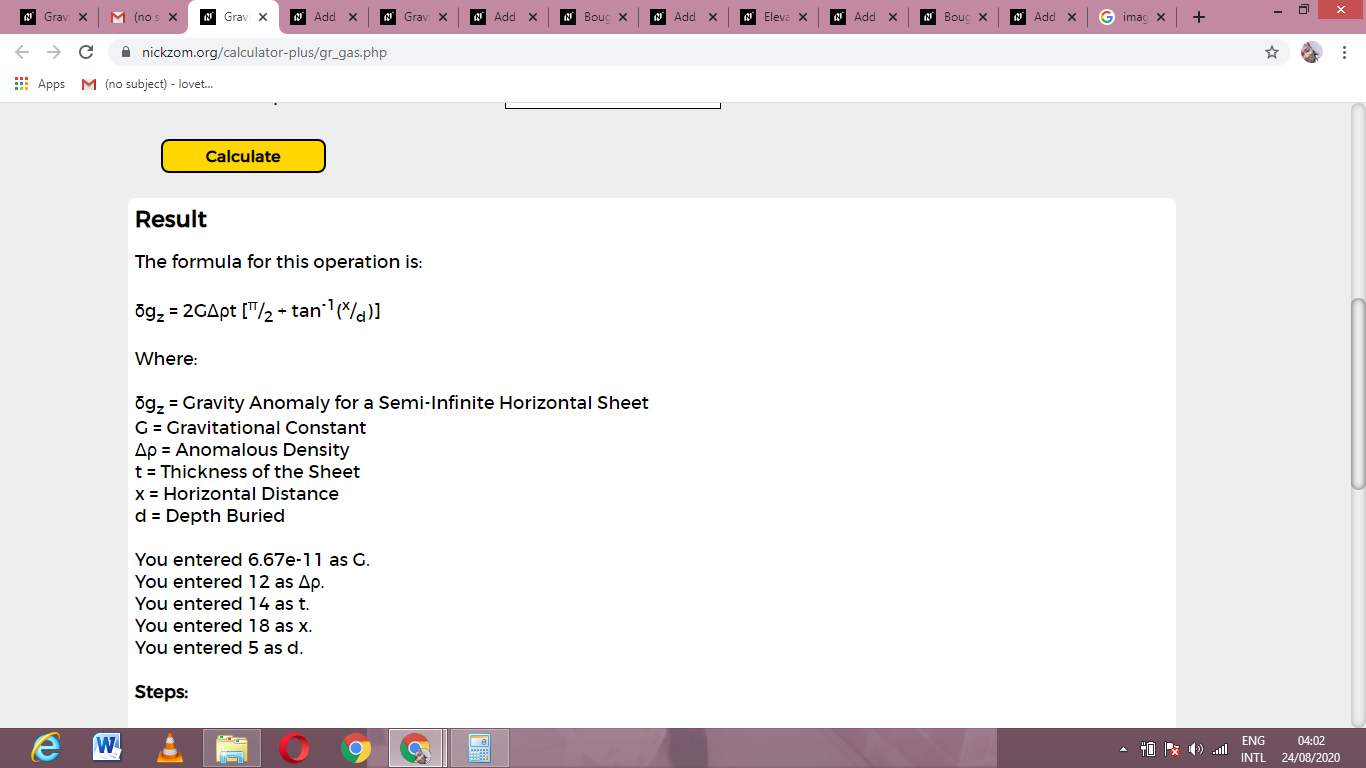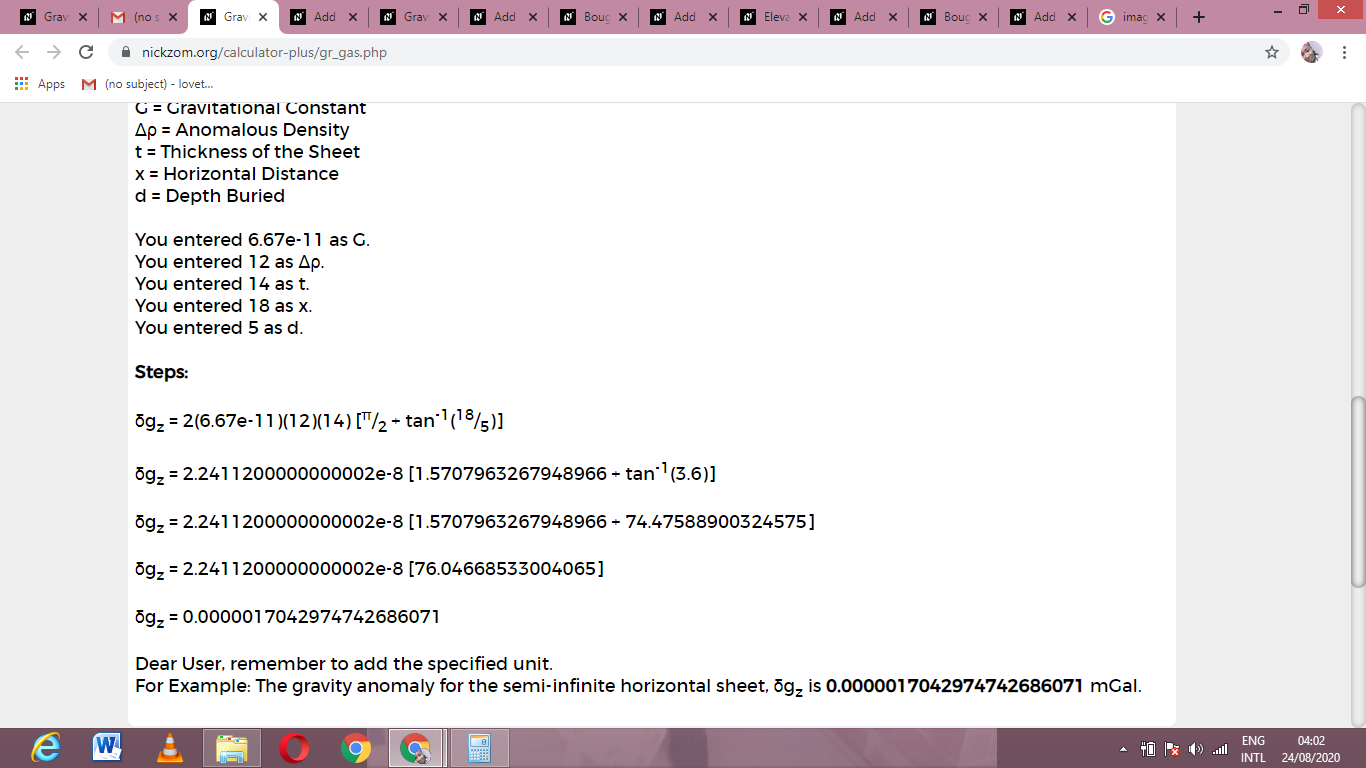As you can see from the screenshot above, Nickzom Calculator– The Calculator Encyclopedia solves for the gravity anomaly for a semi-infinite horizontal sheet and presents the formula, workings and steps too.